Courses

# Test: Automatic Control Systems - 2

## 25 Questions MCQ Test | Test: Automatic Control Systems - 2

Description
This mock test of Test: Automatic Control Systems - 2 for Electrical Engineering (EE) helps you for every Electrical Engineering (EE) entrance exam. This contains 25 Multiple Choice Questions for Electrical Engineering (EE) Test: Automatic Control Systems - 2 (mcq) to study with solutions a complete question bank. The solved questions answers in this Test: Automatic Control Systems - 2 quiz give you a good mix of easy questions and tough questions. Electrical Engineering (EE) students definitely take this Test: Automatic Control Systems - 2 exercise for a better result in the exam. You can find other Test: Automatic Control Systems - 2 extra questions, long questions & short questions for Electrical Engineering (EE) on EduRev as well by searching above.
QUESTION: 1

Solution:
QUESTION: 2

Solution:
QUESTION: 3

### If the characteristic equation is (λ + 1) (λ + 2) (λ + 3) = 0, the eigen values are

Solution:
QUESTION: 4

If the poles lie in second quadrant, damping ratio is

Solution:
QUESTION: 5

Assertion (A): When performance specifications are given in term of transient response characteristics, root locus method is a suitable method.

Reason (R): When performance specifications in terms of state variables are given, modern control methods can be used.

Solution:
QUESTION: 6

Integral control action

Solution:
QUESTION: 7

If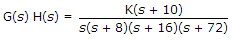, the closed loop pole will be located at s = -12 when K =

Solution:
QUESTION: 8

Robotic manipulator arms mostly use

Solution:
QUESTION: 9

The sensor generally used in incremental optical encoder is

Solution:
QUESTION: 10

In terms of ξ and ωn the settling time of a second order systems for 2% criterion and step input is equal to

Solution:
QUESTION: 11

For type 1 system the velocity lag error is

Solution:
QUESTION: 12

For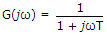as ω approaches infinity, the magnitude approaches zero and phase angle approaches - 90�.

Solution:
QUESTION: 13

In the given figure, the combinations of two non touching loops is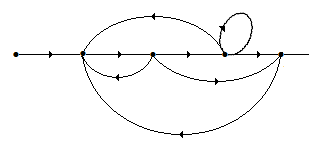Solution:
QUESTION: 14

Nyquist stability criterion uses open loop frequency response characteristics.

Solution:
QUESTION: 15

For any test point s on the real axis, the sum of angular contributions of the complex conjugate poles is

Solution:
QUESTION: 16

For the system in the given figure G varies slightly, the ratio of open loop sensitivity to closed loop sensitivity is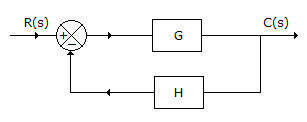Solution:
QUESTION: 17

The loop transfer function of a feedback system is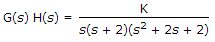. The number of asymptotes of its root loci are

Solution:
QUESTION: 18

The M circle for M = 1 is the

Solution:
QUESTION: 19

Which of the following can act as error detecting devices

1. a pair of potentiometers
2. a pair of synchros
3. a differential transformer
5. a control transformer
Select the answer using the following codes:
Solution:
QUESTION: 20

Assertion (A): If minimum phase system has a gain margin of 8 dB and phase margin of 21º, the system is stable.

Reason (R): For a minimum phase system both GM and PM must be positive for the system to be stable.

Solution:
QUESTION: 21

For 0 < M < 1, the centres of M circle lie to the right of origin.

Solution:
QUESTION: 22

Assertion (A): Integration in time domain is converted into division in s domain

Reason (R):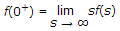Solution:
QUESTION: 23

Assertion (A): In root locus the breakaway and break in points either lie on real axis or occur in complex conjugate pairs.

Reason (R): All root locus asymptotes intersect on real axis.

Solution:
QUESTION: 24

A conditionally stable system is stable for the value of gain between two critical values. It is unstable if

Solution:
QUESTION: 25

Transport lag usually exists in

Solution: